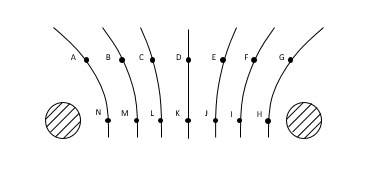# Problem: Two spherical charged conductors placed 6 cm apart produce the equipotential surfaces shown in the figure. The change in potential between each surface is constant, with the 0V line being equidistant from the two charges, and some +V0 at the left-most surface.What is the best comparison of the electric fields at points A and F?A. Both point to the leftB. Both point to the rightC. Field at A points to the right and field at F points to the leftD. Field at A points to the left and field at F points to the rightE. Cannot know without knowing nature of the charges

###### FREE Expert Solution
81% (258 ratings)
###### FREE Expert Solution
81% (258 ratings)View Complete Written Solution
###### Problem Details

Two spherical charged conductors placed 6 cm apart produce the equipotential surfaces shown in the figure. The change in potential between each surface is constant, with the 0V line being equidistant from the two charges, and some +V0 at the left-most surface.

What is the best comparison of the electric fields at points A and F?

A. Both point to the left

B. Both point to the right

C. Field at A points to the right and field at F points to the left

D. Field at A points to the left and field at F points to the right

E. Cannot know without knowing nature of the chargesFrequently Asked Questions

What scientific concept do you need to know in order to solve this problem?

Our tutors have indicated that to solve this problem you will need to apply the Equipotential Surfaces concept. You can view video lessons to learn Equipotential Surfaces. Or if you need more Equipotential Surfaces practice, you can also practice Equipotential Surfaces practice problems.

How long does this problem take to solve?

Our expert Physics tutor, Juan took 3 minutes and 12 seconds to solve this problem. You can follow their steps in the video explanation above.